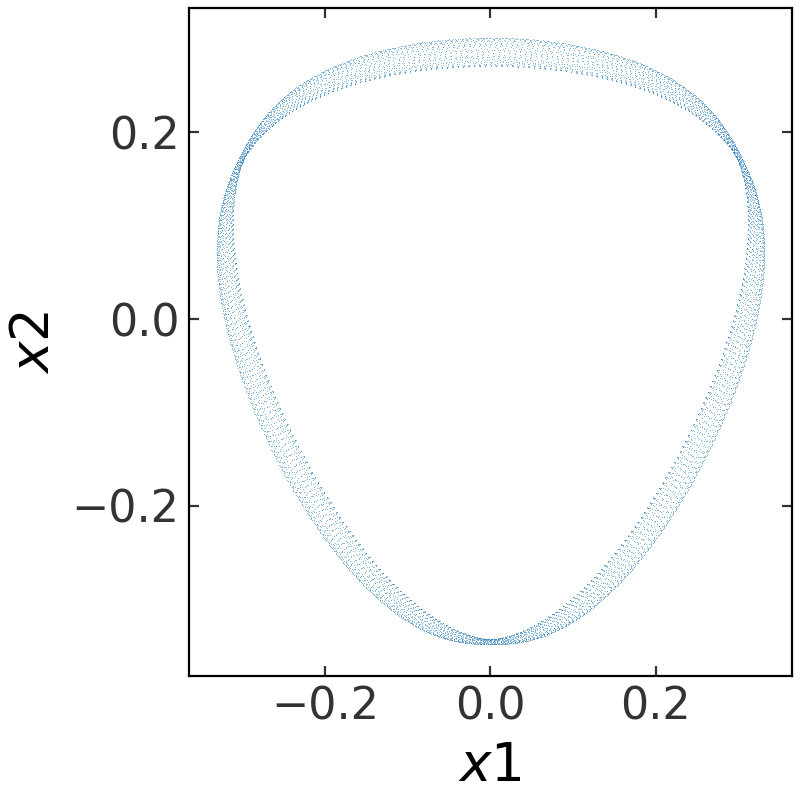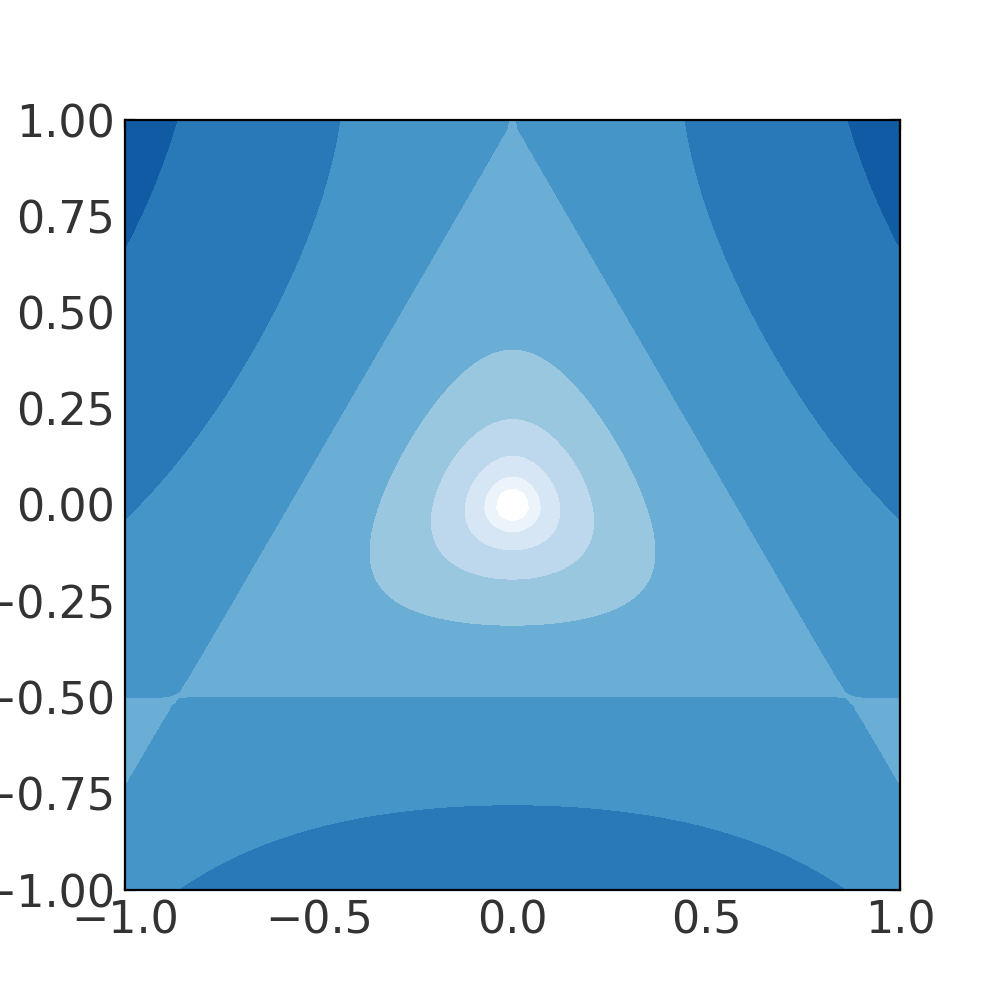# Defining your own potential class¶

## Introduction¶

There are two ways to define a new potential class: with pure-Python, or with C and Cython. The advantage to writing a new class in Cython is that the computations can execute with C-like speeds, however only certain integrators support using this functionality (Leapfrog and DOP853) and it is a bit more complicated to set up the code to build the C+Cython code properly. If you are not familiar with Cython, you probably want to stick to a pure-Python class for initial testing. If there is a potential class that you think should be included, feel free to suggest the new addition as a GitHub issue!

For code blocks below and any pages linked below, we assume the following imports have already been executed:

>>> import numpy as np
>>> import gala.potential as gp
>>> import gala.dynamics as gd


### Implementing a new potential with Python¶

New Python potentials are implemented by subclassing PotentialBase and defining functions that compute (at minimum) the energy and gradient of the potential. We will work through an example below for adding the Henon-Heiles potential.

The expression for the potential is:

$\Phi(x,y) = \frac{1}{2}(x^2 + y^2) + A\,(x^2 y - \frac{y^3}{3})$

With this parametrization, there is only one free parameter (A), and the potential is two-dimensional.

At minimum, the subclass must implement:

• __init__()

• _energy()

• _gradient()

The _energy() method will compute the potential energy at a given position and time. The _gradient() computes the gradient of the potential. Both of these methods must accept two arguments: a position, and a time. These internal methods are then called by the PotentialBase superclass methods energy() and gradient(). The superclass methods convert the input position to an array in the unit system of the potetial for fast evalutaion. The input to these superclass methods can be Quantity objects, PhaseSpacePosition objects, or ndarray.

Because this potential has a free parameter, the __init__ method must accept a parameter argument and store this in the parameters dictionary attribute (a required attribute of any subclass). Let’s write it out, then work through what each piece means in detail:

>>> class CustomHenonHeilesPotential(gp.PotentialBase):
...     A = gp.PotentialParameter("A")
...     ndim = 2
...
...     def _energy(self, xy, t):
...         A = self.parameters['A'].value
...         x,y = xy.T
...         return 0.5*(x**2 + y**2) + A*(x**2*y - y**3/3)
...
...         A = self.parameters['A'].value
...         x,y = xy.T
...
...         grad[:,0] = x + 2*A*x*y
...         grad[:,1] = y + A*(x**2 - y**2)


The internal energy and gradient methods compute the numerical value and gradient of the potential. The __init__ method must take a single argument, A, and store this to a paremeter dictionary. The expected shape of the position array (xy) passed to the internal _energy() and _gradient() methods is always 2-dimensional with shape (n_points, n_dim) where n_points >= 1 and n_dim must match the dimensionality of the potential specified in the initializer. Note that this is different from the shape expected when calling the actual methods energy() and gradient()!

Let’s now create an instance of the class and see how it works. For now, let’s pass in None for the unit system to designate that we’ll work with dimensionless quantities:

>>> pot = CustomHenonHeilesPotential(A=1., units=None)


That’s it! Now we have a fully-fledged potential object. For example, we can integrate an orbit in this potential:

>>> w0 = gd.PhaseSpacePosition(pos=[0.,0.3],
...                            vel=[0.38,0.])
>>> orbit = gp.Hamiltonian(pot).integrate_orbit(w0, dt=0.05, n_steps=10000)
>>> fig = orbit.plot(marker=',', linestyle='none', alpha=0.5)Or, we could create a contour plot of equipotentials:

>>> grid = np.linspace(-1., 1., 100)
>>> from matplotlib import colors
>>> import matplotlib.pyplot as plt
>>> fig, ax = plt.subplots(1, 1, figsize=(5,5))
>>> fig = pot.plot_contours(grid=(grid,grid),
...                         levels=np.logspace(-3, 1, 10),
...                         norm=colors.LogNorm(),
...                         cmap='Blues', ax=ax)### Adding a custom potential with Cython¶

Adding a new Cython potential class is a little more involved as it requires writing C-code and setting it up properly to compile when the code is built. For this example, we’ll work through how to define a new C-implemented potential class representation of a Keplerian (point-mass) potential. Because this example requires using Cython to build code, we provide a separate demo GitHub repository with an implementation of this potential with a demonstration of a build system that successfully sets up the code.

New Cython potentials are implemented by subclassing CPotentialBase, subclassing CPotentialWrapper, and defining C functions that compute (at minimum) the energy and gradient of the potential. This requires creating (at minimum) a Cython file (.pyx), a C header file (.h), and a C source file (.c).